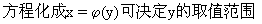由方程画出曲线的基本方法是描点法.为了用较少的点画出较准确的图形，要对方程进行讨论：

(1)曲线的范围：把方程f(x,y)=0化成y=f(x)可决定x的取值范围，把(2)曲线的对称性：如果用-y代替方程f(x,y)=0中的y，方程f(x,y)=0不变，则曲线关于x轴对称；如果用-x代替方程f(x,y)=0中的x，方程f(x,y)=0不变，则曲线关于y轴对称；如果用-x-y分别代替方程f(x,y)=0中的xy，方程f(x,y)=0不变，则曲线关于原点对称．

(3)曲线与坐标轴的交点：在方程f(x,y)=0中，令y=0，可求出曲线与x轴的交点；在方程f(x,y)=0中，令x=0，可求出曲线与y轴的交点．